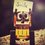# Physics doubt!

If $$\vec{E}$$ is given by$$\vec{E} = y \hat{i} +x \hat{j}$$ , then find the the potential $$V_{(x,y)}$$ if $$V_{∞}$$ is 10 $$V$$.

I did

$\int_{10}^{V_{(x,y)}} dV= -\int_{∞}^{(x,y)} (y \hat{i} +x \hat{j}).(dx \hat{i} +dy \hat{j})$

Substitute $z=xy$

$V_{(x,y)} - 10 = [z]^{xy} _ ∞$

$\color{#D61F06}{V_{(x,y)} - 10 = xy - ∞}$

Why that happened

 - Issue resolved , thanks soumo for help.Note by Kushal Patankar
6 years, 2 months ago

This discussion board is a place to discuss our Daily Challenges and the math and science related to those challenges. Explanations are more than just a solution — they should explain the steps and thinking strategies that you used to obtain the solution. Comments should further the discussion of math and science.

When posting on Brilliant:

• Use the emojis to react to an explanation, whether you're congratulating a job well done , or just really confused .
• Ask specific questions about the challenge or the steps in somebody's explanation. Well-posed questions can add a lot to the discussion, but posting "I don't understand!" doesn't help anyone.
• Try to contribute something new to the discussion, whether it is an extension, generalization or other idea related to the challenge.

MarkdownAppears as
*italics* or _italics_ italics
**bold** or __bold__ bold
- bulleted- list
• bulleted
• list
1. numbered2. list
1. numbered
2. list
Note: you must add a full line of space before and after lists for them to show up correctly
paragraph 1paragraph 2

paragraph 1

paragraph 2

[example link](https://brilliant.org)example link
> This is a quote
This is a quote
    # I indented these lines
# 4 spaces, and now they show
# up as a code block.

print "hello world"
# I indented these lines
# 4 spaces, and now they show
# up as a code block.

print "hello world"
MathAppears as
Remember to wrap math in $$ ... $$ or $ ... $ to ensure proper formatting.
2 \times 3 $2 \times 3$
2^{34} $2^{34}$
a_{i-1} $a_{i-1}$
\frac{2}{3} $\frac{2}{3}$
\sqrt{2} $\sqrt{2}$
\sum_{i=1}^3 $\sum_{i=1}^3$
\sin \theta $\sin \theta$
\boxed{123} $\boxed{123}$

Sort by:

Here Electric field at infity is infinite , so you can't take potential reference as 10 volt at that place (region) . Since E.F here has trajectory of hyperbola , which is unbound at very very very large distance from origin , So potential at infinite being 10 volt is meaningless .

- 6 years, 2 months ago

I didn't got why or how it is meaningless

- 6 years, 2 months ago

• Did you create this problem?

• I want you to spot a fallacy (if it's there) in the following :

${ V }_{ \left( A \right) }-{ V }_{ \left( B \right) }=-\int _{ B }^{ A }{ E.dr } \\ { V }_{ \left( A \right) }-{ V }_{ \left( \infty \right) }=-\int _{ \infty }^{ A }{ E.dr } \\ { V }_{ \left( A \right) }=-E.A+E.\infty$

I have assumed $\displaystyle { V }_{ \infty }=0$ and $E$ to be constant.

So, what is wrong? Is even anything wrong?

• We know that $\displaystyle { V }_{ \left( A \right) }-{ V }_{ \left( B \right) }=-\int _{ B }^{ A }{ E.dr }$. To find the electric potential at $A$ we choose $B$ to be a reference point. It is very nice if we choose $B$ such that the electric potential at $B$ is zero. According to your $E$ (electric field), potential is zero at $x=0,y=0$ i.e., at the origin.

• So do we need to find $\displaystyle { V }_{ \left( A \right) }-{ V }_{ \left( \infty \right) }$? or $\displaystyle { V }_{ \left( A \right) }-{ V }_{ \left( 0 \right) }$ i.e. $\displaystyle { V }_{ \left( A \right) }$

Only after making some assumptions, I came across the following approach:

If $\displaystyle { V }_{ \left( A \right) }-{ V }_{ \left( B \right) }=-\int _{ B }^{ A }{ E.dr }$

Then $\displaystyle { V }_{ \left( A \right) }-{ V }_{ \left( 0 \right) }=-\int _{ 0 }^{ A }{ E.dr }$ gives the potential at $A$ w.r.t origin $0,0$.

On the same basis ${ V }_{ \infty }-{ V }_{ \left( 0 \right) }=-\int _{ 0 }^{ A }{ E.dr }$ gives the potential at infinity w.r.t to origin.

And $\displaystyle \left( { V }_{ \left( A \right) }-{ V }_{ \left( 0 \right) } \right) -\left( { V }_{ \infty }-{ V }_{ \left( 0 \right) } \right)$ gives potential at $A$ w.r.t infinity.

• Now let me return to the very first question: 'Did you create this problem?'. If yes, then I think you can polish it by providing a relation between $x$ & $y$. I am saying so because only after trying to find the role of $x$ & $y$, I stumbled upon the idea of trying to have $x=0,y=0$.

Hope this helps you to find the answer(s) even if this isn't the answer.

:)

- 6 years, 2 months ago

The problem is that, if I consider any point in x-y plane I get answer of electric potential as -infinity .I am unable to figure out what's the problem.

Why is relation between x and y required?

- 6 years, 2 months ago

The ultimate aim is to find the potential at a point w.r.t another point where we can reasonably take the potential to be zero. This reference point need not necessarily be infinity.

I wanted you to spot the fallacy in second point of my previous comment. That way we always get potential at any point to be infinity. That approach is wrong.

Did you try this $\displaystyle \left( { V }_{ \left( A \right) }-{ V }_{ \left( 0 \right) } \right) -\left( { V }_{ \infty }-{ V }_{ \left( 0 \right) } \right)$?

- 6 years, 2 months ago